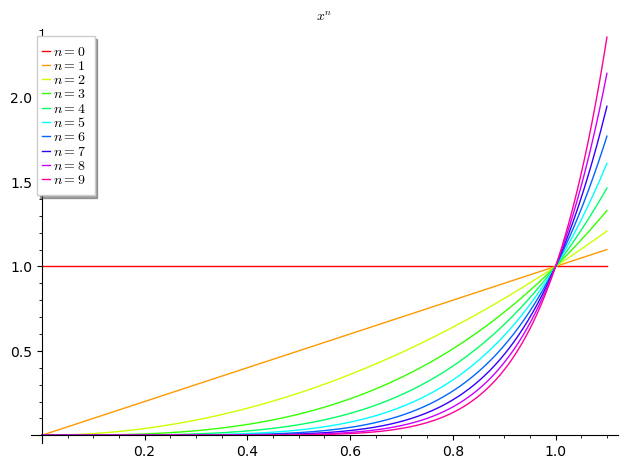# TeX label and running list-variable simultaneously in a plot legend (or plot title)

I want to use a [... for n in ...] to generate plots. How can I combine in a legend and/or title, TeX with the variable that runs (here $n$) to generate the list of plots? Minimal example:

some_plots=[plot(x^n,(x,0,1.1),title=r'$x^n$ where $n$ takes the value in the box
(format is not nice, though)',
legend_label=n, rgbcolor=hue(.1*n)) for n in range(0,10)]
sum(some_plots[s] for s in range(0,10))


which shows a box with colors, and, separately the title. How can I add $n$ to the box? If I combine TeX and the variable (like $x^n$=n) I get errors. (I cannot upload yet pictures, which would make the question very clear)

edit retag close merge delete

Sort by » oldest newest most voted

Is this :

sage: some_plots=[plot(x^u, (0,1.1), title=r"$x^n$", legend_label="$n=%d$"%u, rgbcolor=hue(0.1*u)) for u in range(0,10)]
sage: sum(some_plots)what you mean (up to legend placement, which I'm too lazy to fine tune...) ?

more

Google "string splicing" for Python abilities in this department (which are multiple...).